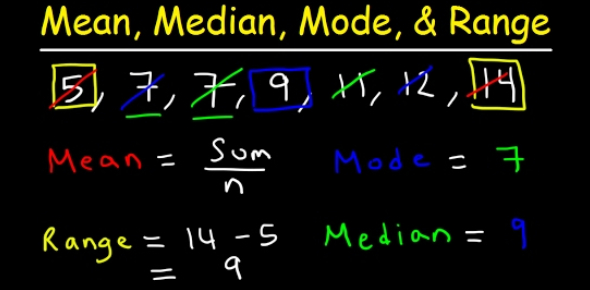# Statistic Quiz: Mean, Median, Mode, And Range

9 Questions | Total Attempts: 28422SettingsStatistics has a wide area of applications, for example, data science and analytics. This is just a simple test for finding out the mean, median, mode and range. If you can score 80% or better than you are excellent. Good luck. :D

• 1.
Find the mean in the following numbers: 19, 21, 18, 17, 18, 22, 46
• A.

23

• B.

16

• C.

19

• D.

500

• E.

None of the above

• 2.
What is the median in the following numbers: 19, 21, 18, 17, 18, 22, 46
• A.

15

• B.

19

• C.

21

• D.

22

• E.

None of the above

• 3.
Find the mean in the following numbers: 9, 8, 15, 8, 20
• A.

12

• B.

16

• C.

15

• D.

10

• E.

None of the above

• 4.
Find the median in the following numbers: 9, 8, 15, 8, 20
• A.

20

• B.

15

• C.

9

• D.

22

• E.

None of the above

• 5.
Find the mode in the following numbers: 9, 8, 15, 8, 20
• A.

15

• B.

8

• C.

9

• D.

20

• E.

None of the above

• 6.
Find the range in the following numbers: 9, 8, 15, 8, 20
• A.

12

• B.

900

• C.

14

• D.

15

• E.

None of the above

• 7.
Find the mean in the following numbers: 36, 38, 33, 34, 32, 30, 34, 35
• A.

54

• B.

34

• C.

74

• D.

44

• E.

None of the above

• 8.
Find the median in the following numbers: 36, 38, 33, 34, 32, 30, 34, 35
• A.

34

• B.

55

• C.

23

• D.

45

• E.

None of the above

• 9.
Find the mode in the following numbers: 36, 38, 33, 34, 32, 30, 34, 35
• A.

34

• B.

38

• C.

36

• D.

30

• E.

None of the above

Related TopicsBack to top